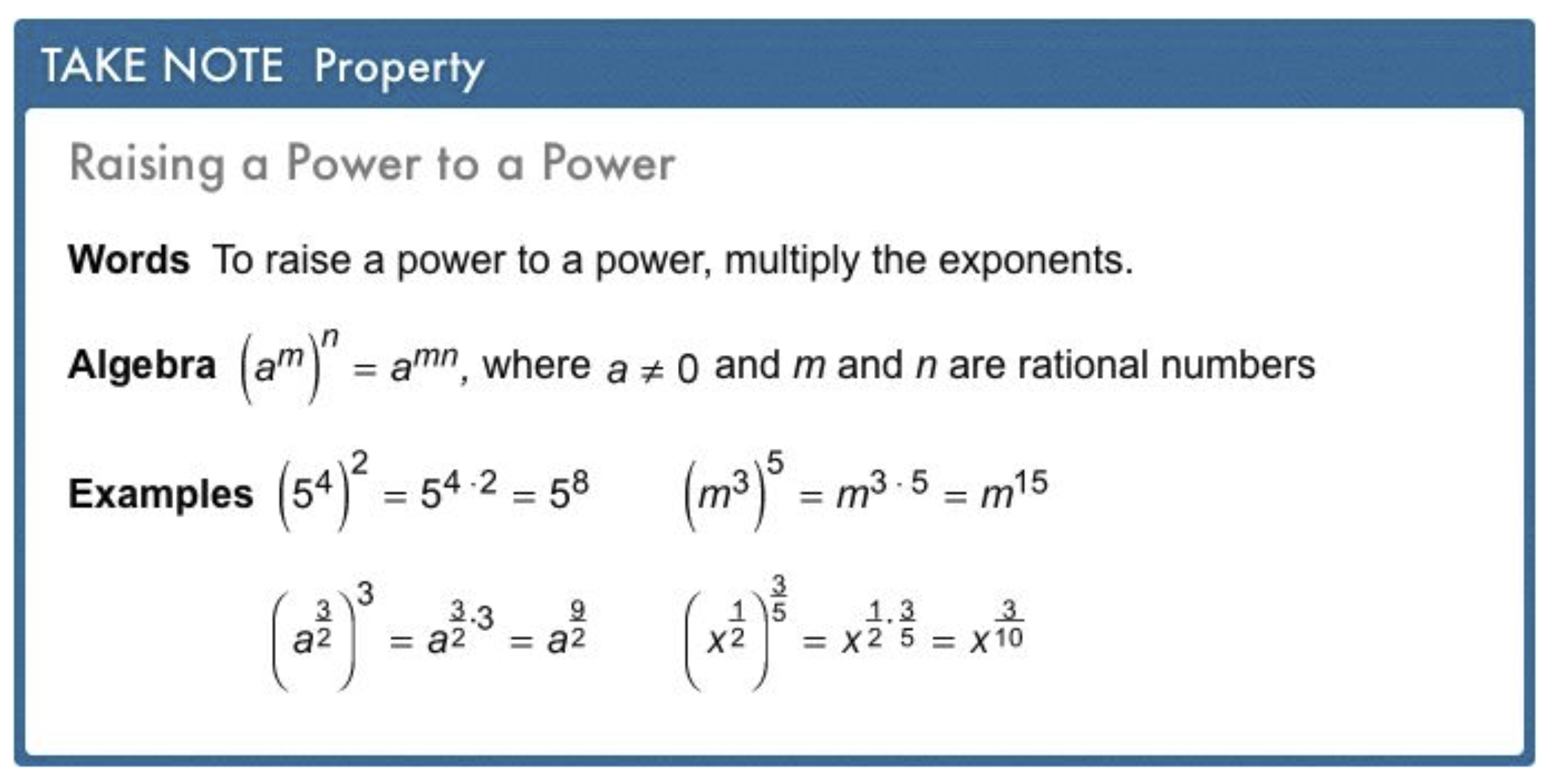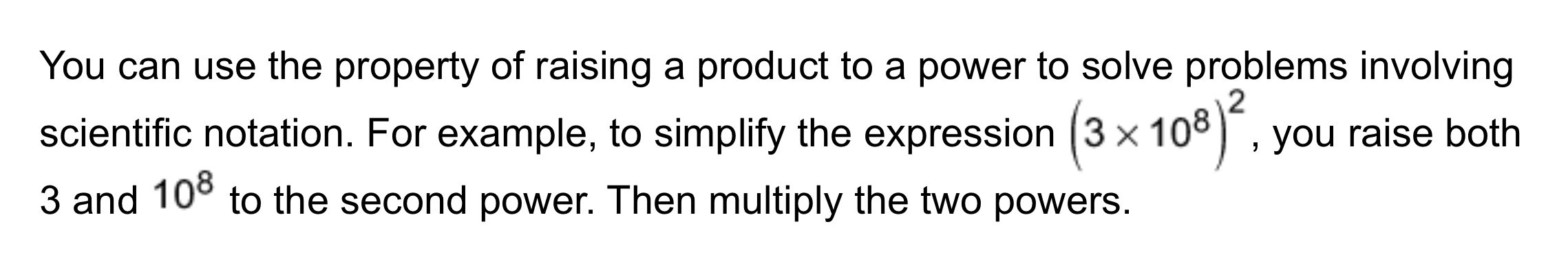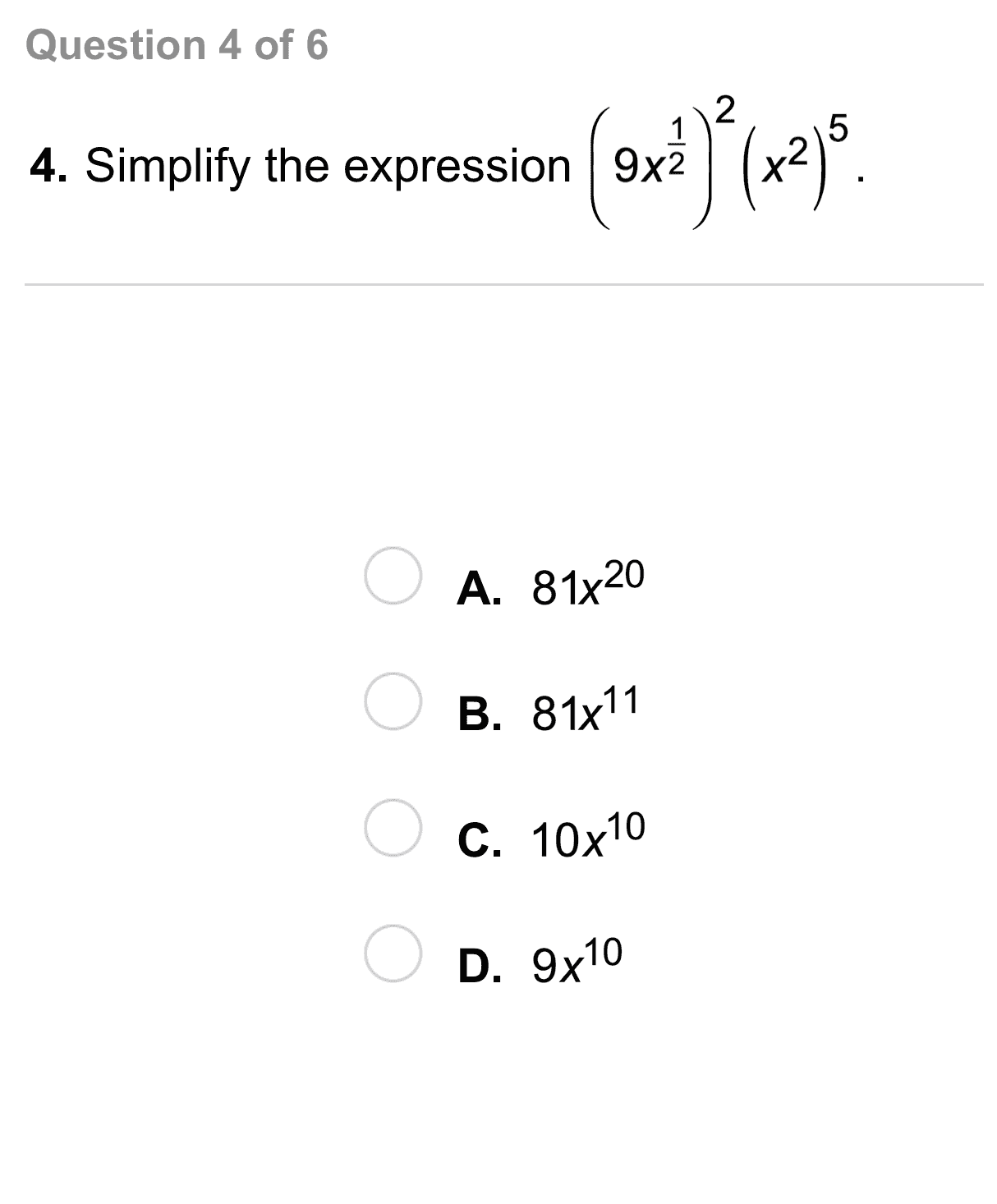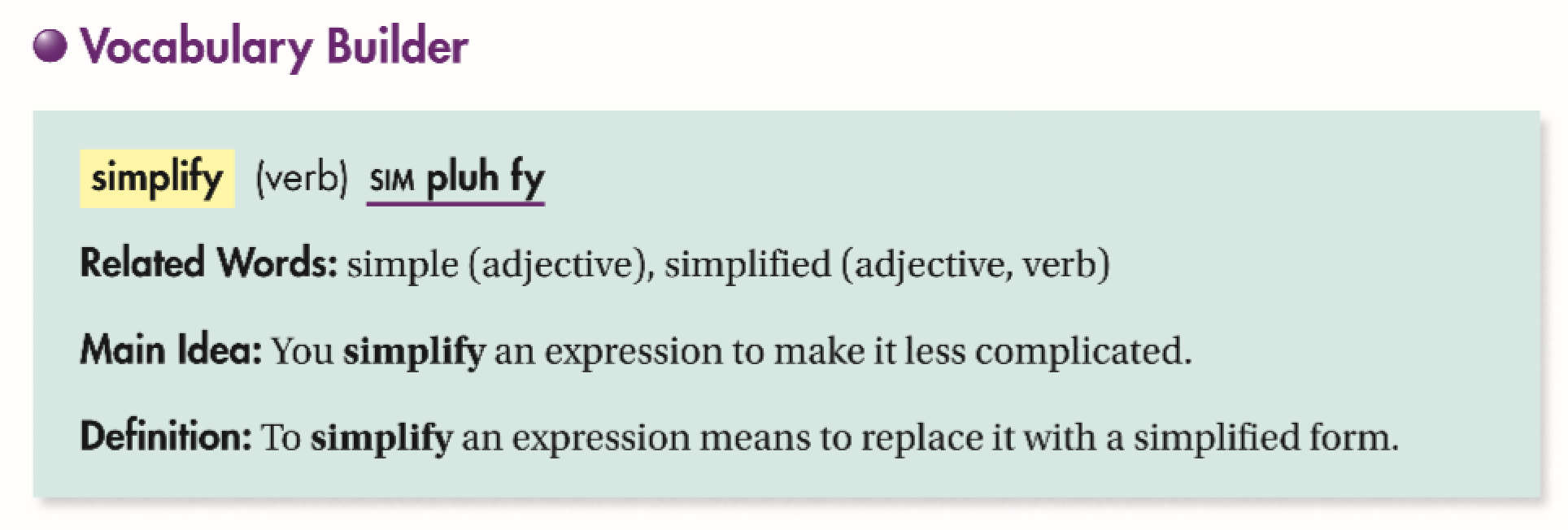Algebra 1 7-3 Complete Lesson: More Multiplication Properties of Exponents
starstarstarstarstarstarstarstarstarstar
5 (1 rating)
by Matthew Richardson
| 40 Questions
Note from the author:
A complete formative lesson with embedded slideshow, mini lecture screencasts, checks for understanding, practice items, mixed review, and reflection. I create these assignments to supplement each lesson of Pearson's Common Core Edition Algebra 1, Algebra 2, and Geometry courses. See also mathquest.net and twitter.com/mathquestEDU.The outlined content above was added from outside of Formative.1
1
10 pts
Solve It! The radius of a bubble made by the bubble machine on the right is 2.5 times as large as the radius of a bubble made by the bubble machine on the left. What is the volume of a bubble made by the machine on the right?2
2
10 pts
Problem 1 Got It?
A
B
C
D3
3
10 pts
Problem 1 Got It?
A
B
C
D4
4
10 pts
Problem 1 Got It?
A
B
C
D5
5
10 pts
Problem 1 Got It?
A
B
C
D6
6
10 pts
Problem 1 Got It?
A
B
C
D7
7
10 pts
Problem 2 Got It?
A
B
C
D8
8
10 pts
Problem 2 Got It?
A
B
C
D9
9
10 pts
Problem 2 Got It?
A
B
C
D10
10
10 pts
Problem 3 Got It?
A
B
C
D11
11
10 pts
Problem 3 Got It?
A
B
C
D12
12
10 pts
Problem 3 Got It?
A
B
C
D13
13
10 pts
Problem 4 Got It?
A
B
C
D14
14
10 pts
Problem 4 Got It?
A
B
C
D15
15
10 pts
Problem 4 Got It?
A
B
C
D16
16
10 pts
Problem 5 Got It?
A
B
C
D17
17
10 pts
A
B
C
D18
18
10 pts
A
B
C
D19
19
10 pts
A
B
C
D20
20
10 pts
A
B
C
D21
21
10 pts
A
B
C
D22
22
10 pts
A
B
C
D23
10 pts
Vocabulary: Compare and contrast the property for raising a power to a power and the property for multiplying powers with the same base.
24
10 pts
Error Analysis: Randy simplified the expression like this:
Ralphie simplified the expression like this:
Who is correct? Explain.
25
10 pts
Open-Ended: Write four different expressions that are equivalent to this expression.26
10 pts
Review Lesson 7-2: Simplify the expression.

27
10 pts
Preview Lesson 7-4: Write the fraction in simplest form.
28
10 pts
Review Lesson 7-2: Simpilify the expression.

29
10 pts
Review Lesson 7-2: Simpilify the expression.

30
10 pts
Review Lesson 5-1: Match the slopes with the lines passing through each given pair of points.
• -3/2
• 6
• -3/4
• -9
• (0, 3), (4, 0)
• (2, -5), (3, 1)
• (-3, 6), (1, 0)
• (0, 0), (1, -9)
31
10 pts
Preview Lesson 7-4: Write the fraction in simplest form.
32
10 pts
Preview Lesson 7-4: Write the fraction in simplest form.
33
10 pts
Preview Lesson 7-4: Write the fraction in simplest form.34
10 pts
Preview Lesson 7-4: Write the fraction in simplest form.
35
10 pts
Vocabulary Review: Classify each equation based on the property of addition it demonstrates.
• 3 + 5 = 5 + 3
• (2 + 3) + 4 = 2 + (3 + 4)
• 7 + 0 = 736
10 pts
Use Your Vocabulary: Match each expression on the left with its simplified form on the right.

37
10 pts
Use Your Vocabulary: Complete the sentence with the appropriate word from the list: simplify, simplified, and simplifying. Enter only the word.

The first step in __?__ the expression below is to subtract within parentheses.

38
10 pts
Use Your Vocabulary: Complete the sentence with the appropriate word from the list: simplify, simplified, and simplifying. Enter only the word.

The __?__ form of the expression below is 18h².
39
100 pts
Notes: Take a clear picture or screenshot of your Cornell notes for this lesson. Upload it to the canvas. Zoom and pan as needed.